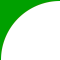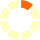# Ohio's Learning Standards: 3.MD.2

## Measure and estimate liquid volumes and masses of objects using standard units of grams, kilograms, and liters. Add, subtract, multiply, or divide whole numbers to solve one-step word problems involving masses or volumes that are given in the same units, e.g., by using drawings (such as a beaker with a measurement scale) to represent the problem. Excludes multiplicative comparison problems involving notions of "times as much"; see Table 2, page 95.